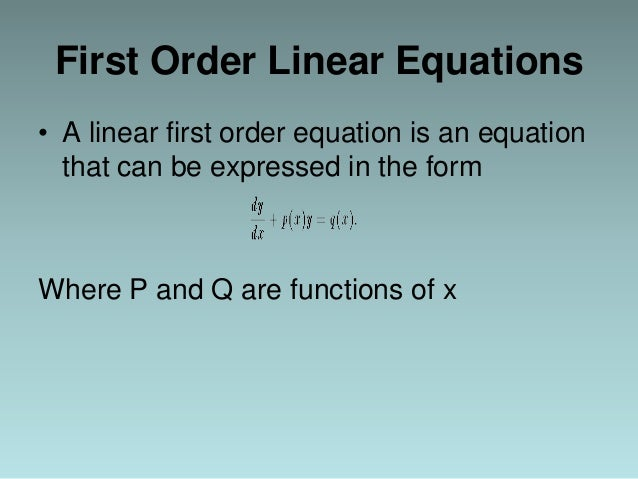# Linear Differential Equations

Contents

##Phenomena as diverse as the motion of the planets, the spread of a disease, and the oscillations of a suspension bridge are governed by differential equations. This course is an introduction to the mathematical theory of ordinary differential equations and follows a modern dynamical systems approach. In particular, equations are analyzed using qualitative, numerical, and if possible, symbolic techniques.

MATH226 is essentially the edX equivalent of MA226; a one-semester course in ordinary differential equations taken by more than 500 students per year at Boston University. It is divided into three parts. MATH226.2x is the second part.

For additional information on obtaining credit through the ACE Alternative Credit Project, please visithere.

Hide

### What you’ll learn

• How to take advantage of techniques to model linear equations
• How to analyze equations with two dependent variables using qualitative, numerical, and symbolic techniques
• Forced second-order linear equations and related phenomena such as beats and resonance

### Pursue a Verified Certificate to highlight the knowledge and skills you gain (\$49)

• #### Official and Verified

Receive an instructor-signed certificate with the institution’s logo to verify your achievement and increase your job prospects